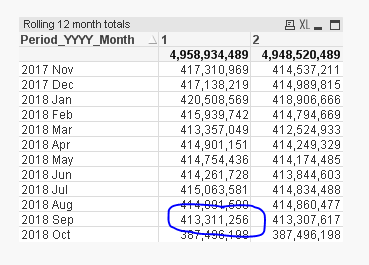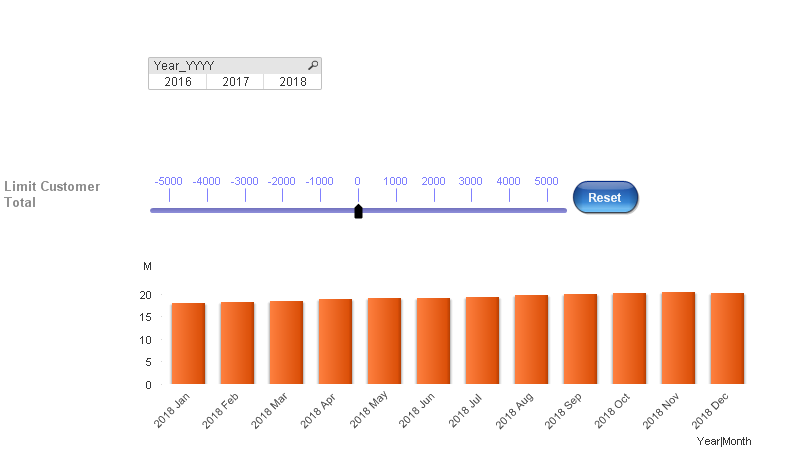# New to QlikView

Discussion board where members can get started with QlikView.

Contributor III

## Re: Rolling 12 months calc, not showing correct figures

Just some observations:

- In Sunny's table document, you can see that 2017 Oct is the first month for which the value in  is different from the value in , which is why for 2018 Oct your total value was fine

- When you select 2018 Sep in your original file, the total value IS 413,311,256

- When you select 2017 Oct - 2018 Sep in Sunny's document, the values for each month​ the selected months change and become the same as in your image (and the total value adds up to the right amount)

- When you add 1 (ignore selections) to every set analysis in your expression, the values (I think) are correct (or at least, the same as in your image) I thought this worked, but I was wrong

Don't know if any of this helps, just thought I'd put it out there.MVP

## Re: Rolling 12 months calc, not showing correct figures

I get this and you can get this result if you use this expression...

=Sum({<[Period_Sort] = {">=\$(=max([Period_Sort])-11)<=\$(=max([Period_Sort]))"}, Month, Year_YYYY, Quarter_QQ, Period_YYYY_Month>}Aggr(RangeSum(Above(Sum({<[Period_Sort], Month, Year_YYYY, Quarter_QQ, Period_YYYY_Month>} Revenue), 0, 12)), ([Period_Sort],(NUMERIC, ASCENDING))))

Right?Contributor

## Re: Rolling 12 months calc, not showing correct figures

Hi Sunny, many thanks for your reply

Yes the penny has dropped and I get what you mean with the Client_Name, within the calculation.

Basically the client has to appear in every 12 month total, for the numbers to agree.

So in summary, It only takes the client_Name within the first 12 months as it's list for the rest.

Will have to think of another idea, to include all clients with those variables.

any suggestions welcome?MVP

## Re: Rolling 12 months calc, not showing correct figures

I guess I am confused as to what you are looking to do. Can we take a step back (and assume me to be completely dumb for a minute) and explain what exactly are you trying to do?

Contributor

## Re: Rolling 12 months calc, not showing correct figures

you are far from dumb Sunny my friendRight so the calculation you stated works; in that, you get each of those 12 categories showing you the total for that current month plus the previous 11 (so basically a rolling 12 month total for each category).

However, this falls over when you put the client_name within the calculation (as we've seen, gives different totals due to not having all clients)

I require this as per my attached qvw, where i have the min and max variables within a slider (so the user can remove client totals of their choice)

So in summary, I require 12 categories showing 12 month totals, together with the min and max variables at client level.

Simple huh haha

Thanks for your any advice.

Contributor

## Re: Rolling 12 months calc, not showing correct figures

you are far from dumb Sunny my friendRight so the calculation you stated works; in that, you get each of those 12 categories showing you the total for that current month plus the previous 11 (so basically a rolling 12 month total for each category).

However, this falls over when you put the client_name within the calculation (as we've seen, gives different totals due to not having all clients)

I require this as per my attached qvw, where i have the min and max variables within a slider (so the user can remove client totals of their choice)

So in summary, I require 12 categories showing 12 month totals, together with the min and max variables at client level.

Simple huh haha

Thanks for your any advice.MVP

## Re: Rolling 12 months calc, not showing correct figures

Can you check if this is what you want?

=RangeSum(Above(Sum({<[Period_Sort], Month, Year_YYYY, Quarter_QQ, Period_YYYY_Month,

Customer={"=round(Sum({<[Period_Sort], Month, Year_YYYY, Quarter_QQ, Period_YYYY_Month>}Revenue))<=\$(vMinLimit)

or

round(Sum({<[Period_Sort], Month, Year_YYYY, Quarter_QQ, Period_YYYY_Month>} Revenue))>=\$(vMaxLimit)"}>} Revenue), 0, 12))

* Avg({<[Period_Sort]={">=\$(=max([Period_Sort])-11)<=\$(=max([Period_Sort]))"},Month= ,Year_YYYY= ,Quarter_QQ= , Period_YYYY_Month=>} 1)If this is right, I might know what is going on and can explain it better. But before I do, it would be nice to know if this is right or not

Contributor

## Re: Rolling 12 months calc, not showing correct figures

fantastic Sunny, this works and is exactly what i require.

the correct figures for each category of 12 months, working with the two variables.

I am now very intrigued as to know what is going on, so explain awayMany thanks

HighlightedMVP

## Re: Rolling 12 months calc, not showing correct figures

The problem was that your inner set analysis check against Client_Name also included set analysis to only look at >Max_Period - 11 <Max_Period. So, the limits were only checked for those revenues which fell within that date range. So, essentially, any customer which was in the limit but didn't have any sales within the date range was excluded. I removed that bit of the set analysis and boom you got what you needed.

Does that make sense?

Contributor

## Re: Rolling 12 months calc, not showing correct figures

Nice one Sunny, completely makes sensethank you for explaining

One more thing, I would like to understand is;

how does the below line of putting average at the beginning and 1 at the end, limit the categories to just 12 in the table?

* Avg({<[Period_Sort]={">=\$(=max([Period_Sort])-11)<=\$(=max([Period_Sort]))"},Month= ,Year_YYYY= ,Quarter_QQ= , Period_YYYY_Month=>} 1)